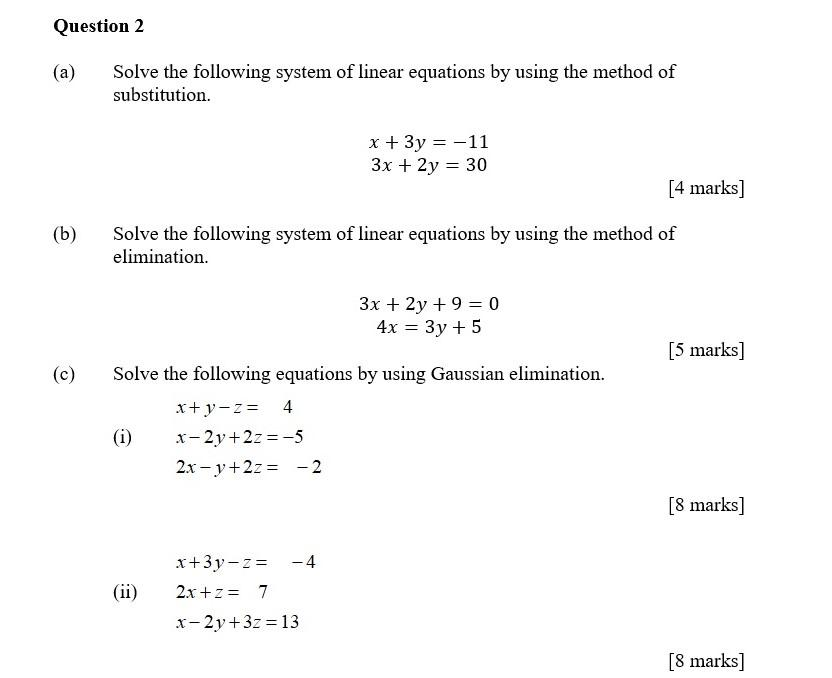Home / Expert Answers / Other Math / a-solve-the-following-system-of-linear-equations-by-using-the-method-of-substitution-begin-a-pa289

# (Solved): a) Solve the following system of linear equations by using the method of substitution. $\begin{a ...a) Solve the following system of linear equations by using the method of substitution. \[ \begin{array}{l} x+3 y=-11 \\ 3 x+2 y=30 \end{array}$ [4 marks] Solve the following system of linear equations by using the method of elimination. $\begin{array}{c} 3 x+2 y+9=0 \\ 4 x=3 y+5 \end{array}$ [5 marks] Solve the following equations by using Gaussian elimination. $x+y-z=4$ (i) $$\quad x-2 y+2 z=-5$$ $2 x-y+2 z=-2$ [8 marks] (ii) $$2 x+z=7$$ $x-2 y+3 z=13$

We have an Answer from Expert